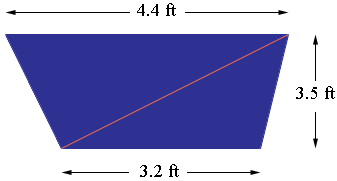SEARCH HOMEMath Central Quandaries & QueriesQuestion from Lorraine, a student: Hey, I'm having problem with an item on our assignment in solid menstruation. Here's the word problem: The vertical end of a trough has the following dimensions: width at top 4.4 ft., width at bottom 3.2 ft., depth 3.5 ft. Find the area at the end of the trough. I hope you could help me solve it. More power and God bless! -LorraineHi Lorraine,

I drew a diagram (not to scale) and added a diagonal to divide the end into two triangles.The area of the end is then the sum of the areas of the two triangles. Can you find the areas of the triangles?

PennyMath Central is supported by the University of Regina and The Pacific Institute for the Mathematical Sciences.